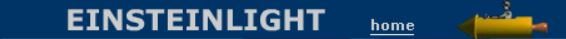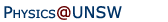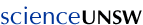Relativity in brief... or in detail..

## E = mc2: The mass defect and binding energies in the nucleus ... and in atoms and molecules.

Summary: The mass of a stable nucleus is less than the sum of its parts by Δm = E/c2, where E is the binding energy. Similarly, the mass of a stable atom or molecule is less than the sum of its parts by Δm = E/c2, where E is the binding energy. The mass defect is evident in nuclear reactions when the masses are measured to several significant figures. The strong force in the nucleus is much larger than the electric force in atoms and molecules. Consequently, the mass defect in atomic and molecular calculations is much smaller and is usually neglected. The difference is quantitative, however, not qualitative. Apart from their strength, there is nothing special about the nuclear forces with regard to the mass defect.

• Mass defect in nuclear reactions
• Mass defect in atomic and molecular reactions
• How did they get those data?
• Measurement of mass defect in atomic and molecular reactions

Einstein's famous equation describes how mass m can be turned into energy E. Conversely, when we do work on something, we provide energy, so we can increase its mass by an amount Δm = E/c2. (The symbol Δ, pronounced 'delta', means 'the change in'.)

First, something about stability. For macroscopic objects, a system is stable if it requires energy to put it into another state. For example, a stick is stable lying on the ground. To lift up one end or the other, we have to give it energy, to do work on it. Conversely, if it is standing vertically on one pointed end it is unstable: it can lose energy by falling over.

### Mass defect in nuclear reactions

Let's start with the atomic nucleus. Happily for us, most of them are stable - they don't disintegrate spontaneously. For example, a deuterium nucleus (which is called a deuteron, symbol d) has just two components, a neutron (n) and a proton (p). When we add an electron (e) to the deuterium nucleus, we get an atom of deuterium (D or 2H).

If we 'pull apart' a deuterium nucleus, we require energy ED. We call this the binding energy of deuterium. When we supply this energy, the mass is increased by Δm = ED/c2. Conversely, when two particles that attract each other come together, they lose energy and their mass decreases.Cartoon: a stable nucleus has a lower mass than the sum of its components. A stable atom or molecule has a lower mass than the sum of its components. In each case, the binding energy E gives rise to a mass defect Δm = E/c2. For nuclei, the mass defect is a very small proportion; for chemical reactions, it is an extremely small proportion. (The effect is grossly exaggerated here.)

Let's take some examples. A convenient unit used for atomic masses is called simply the atomic mass unit (symbol u), which is one twelfth of the mass of an atom of the most prevalent isotope of carbon, 12C. The masses in which we are interested are those of the proton, neutron, deuteron and deuterium:

mp = 1.0073 u
mn = 1.0087 u
md = 2.0141 u

me = 0.00055 u
mD = 2.0146 u

So the sum of the masses of a neutron and a proton is 2.0160 u, which is greater than the mass of a nucleus comprising a neutron and a proton (mD = 2.0141 u). The difference is greater than the mass of an electron. The extra mass represents the energy required to separate the proton and neutron (represented simplistically and exaggerated in the cartoon).

So, if a nucleus is stable, its mass is less than the sum of the masses of its components, and the difference is called the mass defect Δm, if expressed as a mass, or the binding energy E = Δmc2, if expressed as an energy. (We could also go to a smaller scale and consider the fusion of quarks.)

### Mass defect in atomic and molecular reactions

Relativity is not confined to the nucleus. If a molecule or atom is stable, it takes energy to pull its atoms apart. So you could think of the cartoon above as representing an atomic or chemical reaction, as well as a nuclear one. We do work to pull them apart, that work goes into potential energy, and that energy increases the mass of the system. However, the binding energies of molecules are much less than those of nuclei, and so the mass defects involved are smaller. As an example, let's start with one of the most important reactions in the universe*.
Ebinding + H ↔ H+ + e

This gives a mass defect of 1.47 x 10-8 u. Or, doing the calculation another way, the energy needed to remove an electron from the unexcited hydrogen atom is well known: it’s 13.6 eV. The mass of a proton (i.e. a H+) is about 938 MeV/c2 or 1.007 u. So the mass defect due to the binding energy for this reaction, in atomic mass units, is

(13.6 eV/938 MeV)* 1.007 u = 1.47 x 10–8 u.

### How did they get those data?

But we should ask: how does one measure masses to nine or more significant figures? For H+, He++ and e, one can use charge:mass ratio, because the tools of mass spectrometry and Penning traps are very precise. But they cannot be used for the neutral H atom. And indeed, the CIAAW relies on mass spectrometry data to calculate the atomic mass of hydrogen.

### Measurement of mass defect in atomic and molecular reactions

A typical chemical reaction might involve a nett energy of 30 kJ per mole, or 5 x 10-20 J per molecule. So the change in mass is 5 x 10-37 kg, which is only .0001% of the mass of an electron. These defects are small, so we should need a very precise method of measuring mass (via charge:mass ratio), such as mass spectrometry or a Penning trap, and/or a bigger defect to measure. Precise measurement means that all components in the reaction must be charged, so a simple example would be He++ + e ↔ He+. But we could also make the effect bigger by using a highly charged ion. To take an extreme case (with a bit of bling), consider the reaction Au79+ + e ↔ Au78+. Here we have the advantage that the potential energy of the electron close to that highly charged gold nucleus is extremely negative. Consequently, the mass defect is large: of the same order as the electron mass. (While we're considering gold, it's worth noting that the reason why its colour is different from that of most metals is due to relativistic effects on inner electrons: see Relativistic Quantum Chemistry.)

Studies of highly charged ions are regularly performed because the optical transitions between electron states in these ions can be measured very precisely to produce extremely precise time measurements and to test fundamental theories of physics.

Positronium (an 'atom' formed from an electron and a positron) might seem to be another candidate for measuring a purely electric or chemical binding energy. However, it is not stable enough to allow the precision needed (a few parts per million).

I've been asked: Why would CIAAW assume that relativity is true and use it in their published data? Why would they apply relativity in this calculation of a chemical mass defect? The answer is that relativity is a law that has been tested to high precision and hasn’t failed to match the data. Chemistry, at its fundamental level, is quantum mechanics and electricity, and relativistic quantum electrodynamics is by far the most precise and exact theory in all of science – for some measurements, its precision is better than one part in a trillion. (Like measuring the distance to the moon with mm precision.)

The conclusion: the reason why we typically discuss mass defects in relation to nuclear reactions is that the binding energy in such reactions is large enough to produce a mass defect that can be measured  providing the measurements are extremely precise. The mass defect associated with the electric interactions in atomic and chemical reactions requires even greater precision and is usually too small to be measured directly, so chemists dont usually think about it. However, electrical interactions* do indeed produce mass defects. In principle, a measurement of a reaction, chemical or other, that had a binding energy but no mass defect would disprove relativity. However, relativity works just as well for electric forces as it does for nuclear forces.

But someone asked me 'What if the mass defect really were exactly zero in some chemical reaction with a finite binding energy? What then?' Well in that case, simple: relativity would be wrong, and we'd abandon it and search for a new theory. And we'd have to abandon quantum electrodynamics, too. However, because all experiments to date agree with relativity with very high precision, that new theory would have to give the same predictions as relativity for all the experiments to date, at the same precision. It's difficult to see how the new theory could do this and somehow not predict a mass defect for chemistry. (Read more about the Principle of Correspondance.)

For more discussion, see this link. And to see why the neutron and proton attract, see Why there would be no chemistry without relativity. And the example of mass defect in power stations is here.

* The reaction Ebinding + H ↔ H+ + e is important in cosmology. From when the universe was about three minutes old to roughly 380,000 years old, its relative composition was roughly the same. First, the charged components: lots of electrons, almost as H+, a relatively small fraction of He2+ and an even smaller proportion of lithium ions (Li3+) to make it all electrically neutral. Then the uncharged: lots of photons, neutrinos and dark matter. About 380,000 years ago, expansion had cooled the universe (and its photons, which expanded with it) sufficiently that all the H+ , He2+ and Li3+ could combine with electrons without being immediately re-ionised by energetic photons. Thus the universe was suddenly transparent (Let there be dark!). The radiation left at that time is what we see in the famous pictures of the (now much colder) cosmic microwave background.

One could also argue that the production of H+ ions is one of the most important reactions in chemistry and biochemistry, although in those contexts the ion is usually produced from water rather than from a hydrogen atom.

** If nuclear and electric forces both give mass defects, what about gravity? Well in pinciple yes, but gravity is ~1039 times weaker than electricity, so the mass defect would be extremely small. Further, if I lift up an apple, thereby increasing the potential energy of the earth-apple system, the energy I used was already affecting the gravitational field near the apple, so the calculation would be complicated.Home | Summary | Quiz | Credits School of Physics - The University of New South Wales Sydney, Australia 2052 © School of Physics UNSW 2052 Disclaimer feedback